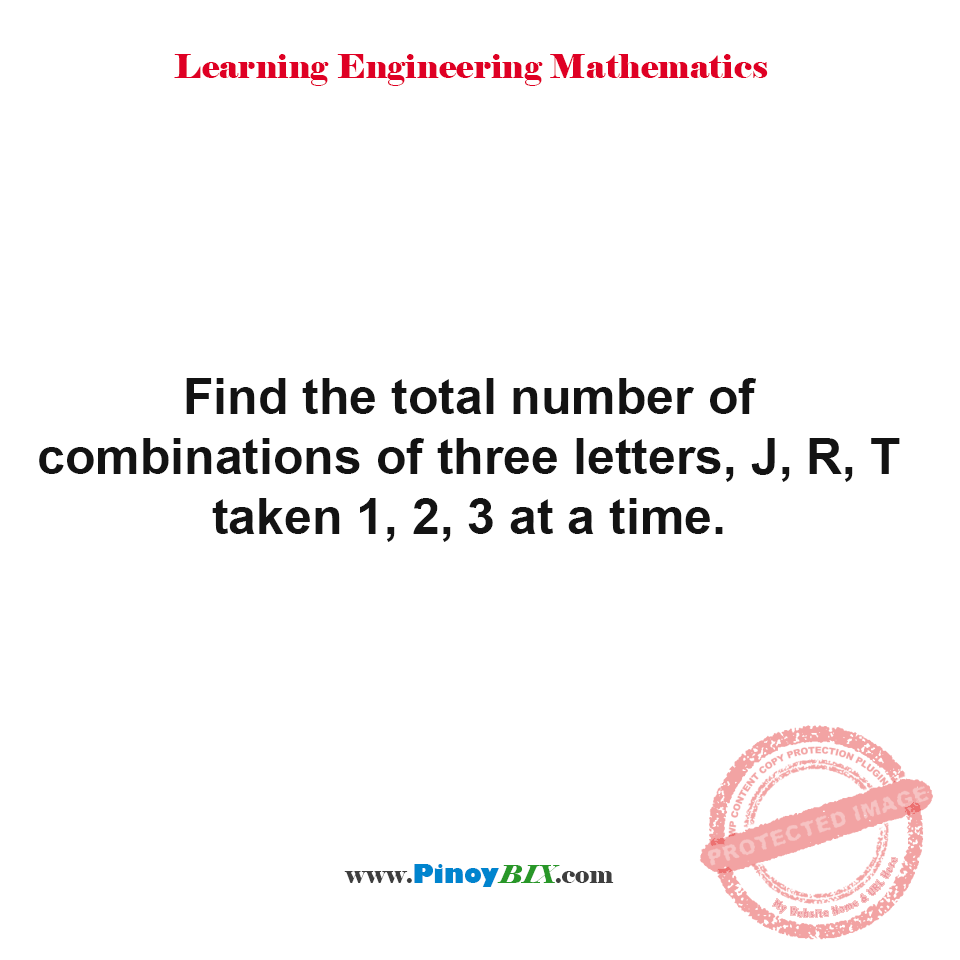# Solution: Find the total number of combinations of three letters at a time.

(Last Updated On: March 12, 2019)#### Problem Statement: EE Board October 1997

Find the total number of combinations of three letters, J, R, T taken 1, 2, 3 at a time.

There are 7 combinations of three letters, J, R, T taken 1, 2, 3 at a time.

### Latest Problem Solving in Venn Diagram, Permutation, Combination and Probability

More Questions in: Venn Diagram, Permutation, Combination and Probability

#### Online Questions and Answers in Venn Diagram, Permutation, Combination and Probability

5.0
01

If you liked this, then please subscribe to our YouTube Channel for engineering video tutorials. You can also find us on Twitter and Facebook.

NEED SUPPORT: LIKE MY PAGE & SHARE